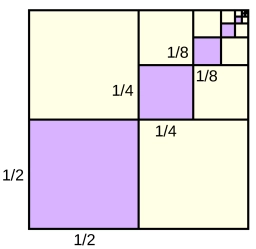# Eduardo

Eduardo wrote a fraction problem by adding 1/4 and then subtracting 2 1/2 in the same pattern, and he got these numbers: 8 5/8, 6 1/8, 6 3/8, 3 7/8
Identify the next two numbers in the pattern

a5 =  4 18 = 33/8
a6 =  1 58 = 13/8

### Step-by-step explanation:Did you find an error or inaccuracy? Feel free to write us. Thank you!

Tips for related online calculators
Need help calculating sum, simplifying, or multiplying fractions? Try our fraction calculator.
Need help with mixed numbers? Try our mixed-number calculator.